This is a guest post by Alan Murray from Computergaga.

There are many ways to round numbers in Excel. These techniques will typically involve formatting the values, or using formulas.

This post will look at 10 rounding functions of Excel, and then look at some formatting techniques.## The ROUND Function

The ROUND function will return a number rounded to a specified number of digits.

You can round a number to the right or left of the decimal point. So whether you want to round to a specific number of decimals, or to the nearest hundredth, the ROUND function can help.

The ROUND function looks like below;

=ROUND(number, num_digits)

The number is the number that you want to round, and then num_digits is how many digits you want to round the value to.

You can enter a positive or negative value for num_digits as shown in the examples below.

A positive value rounds to the right of the decimal point, and a negative value rounds to the left of the decimal point (i.e. to the nearest 10, 100, 1000 etc). You can also enter 0 to simply round to the nearest integer.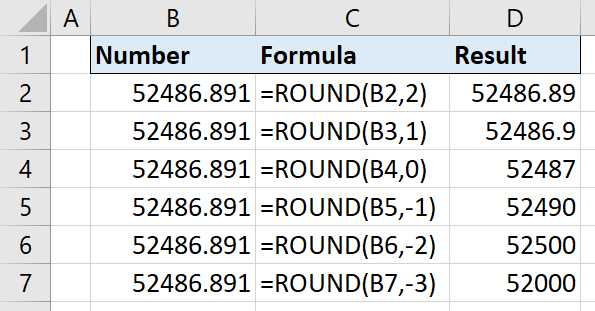The ROUND function will round a number of 5-9 up, and a number of 1-4 down.

## ROUNDUP Function

The ROUNDUP function returns a number rounded up to a specified number of places.

It rounds all numbers from 1 to 9 up.

=ROUNDUP(number, num_digits)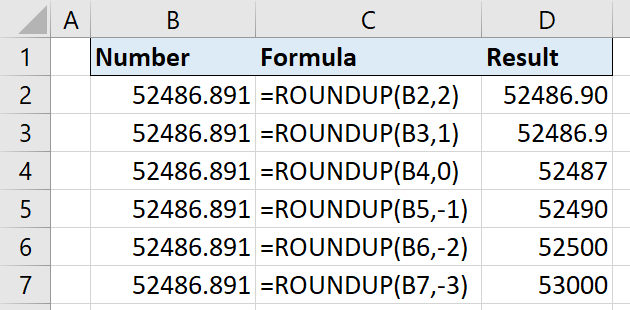## ROUNDDOWN Function

The ROUNDDOWN function returns a number rounded down to a specified number of places.

It rounds all digits from 1 to 9 down.

=ROUNDDOWN(number, num_digits)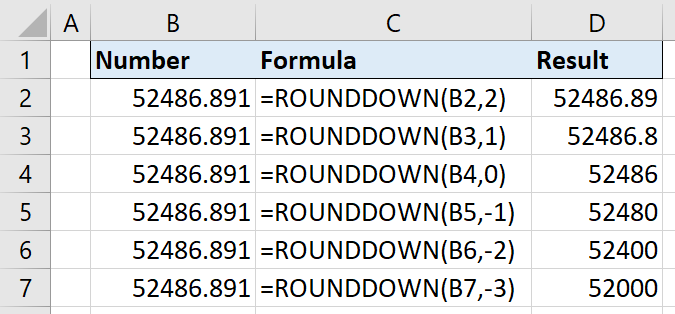## MROUND Function

The MROUND function returns a number rounded to a specified multiple.

For example, this function can be used to;

• Round a price to the nearest 0.05
• Round a time to the nearest 30 minutes.

The MROUND function requires the number you want to round, and the multiple to round it to.

=MROUND(number, multiple)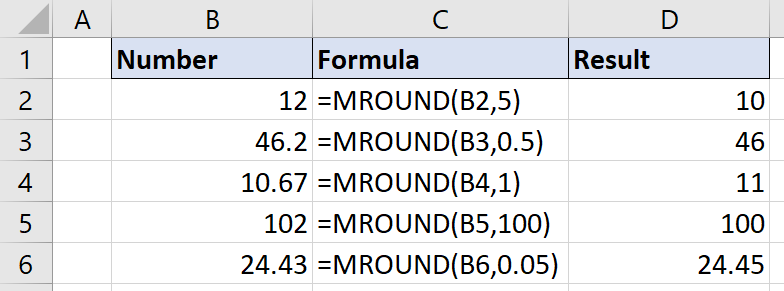Check out this video of the MROUND function being used to round a time to the nearest 15 minutes.

## CEILING Function

The CEILING function will round a number up to the nearest multiple that you specify.

It works like the MROUND function, but ensures that the number is always rounded up.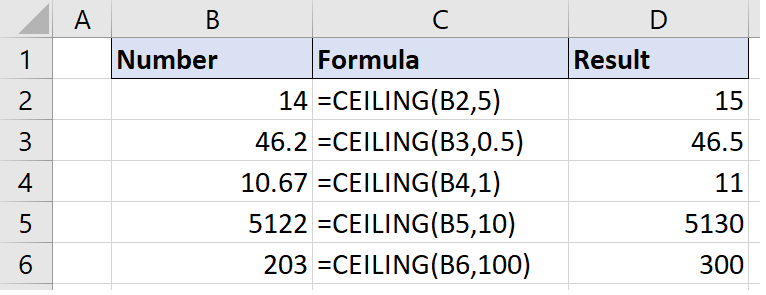## FLOOR Function

The FLOOR function works like the CEILING function but ensures that a number is rounded down to the nearest multiple that you specify.

For example, this function could be used to;

• Round a year down to the nearest decade
• Round a price down to the nearest 0.5.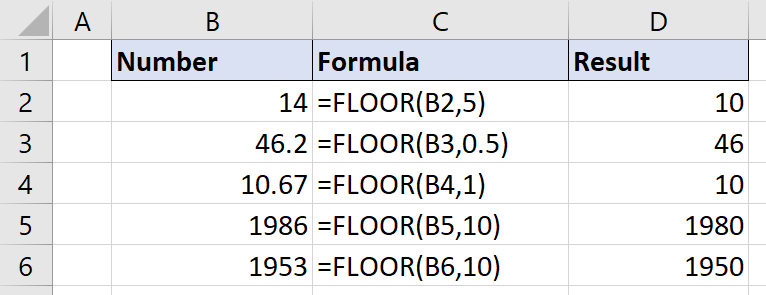## INT Function

The INT function rounds a number down to the nearest integer. This function always rounds down, so a positive number gets smaller, and a negative number becomes more negative.

The INT functions just requires the number to round.

=INT(number)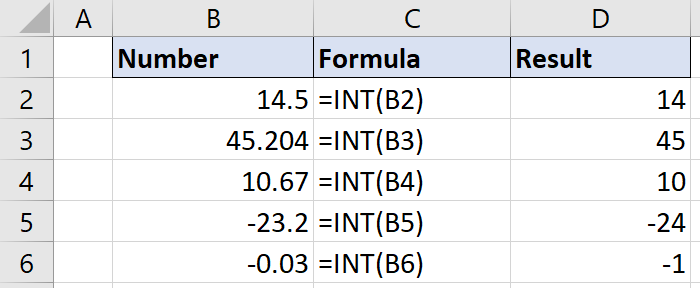There are many interesting uses for the INT function such as to help calculate the days, hours and minutes elapsed between two dates (shown in the video below), or to help create a 5 star rating system.

## TRUNC Function

The TRUNC function extracts the part of a number specified by a given number of digits. Technically this is not a rounding function, but I feel it deserves to be spoken about along with these other functions.

To use the TRUNC function you need to provide the number to truncate, and the number of digits to truncate to.

=TRUNC(number, [num_digits])

Specifying the number of digits to truncate to is optional, and if not specified 0 is used.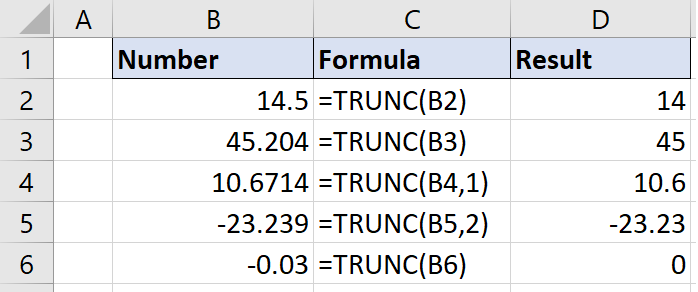The TRUNC function is commonly used to extract the integer part of a number, just like the INT function. However, they differ in the way that TRUNC will simply truncate the integer portion, whilst INT will round down to the nearest integer.

To get only the decimal portion of the number you could do;

=A1-TRUNC(A1)

## ODD Function

The ODD function returns the next odd integer after rounding a number away from zero.

Both positive and negative numbers are rounded away from zero, so positive numbers get bigger, and negative numbers become smaller.

The ODD function just requires the number to round.

=ODD(number)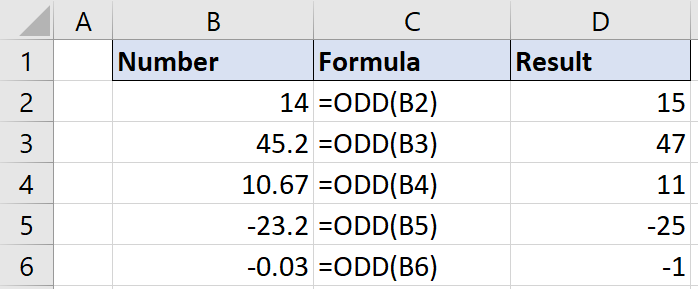## EVEN Function

The EVEN function works in the same way as ODD, but returns the next even integer after rounding away from zero.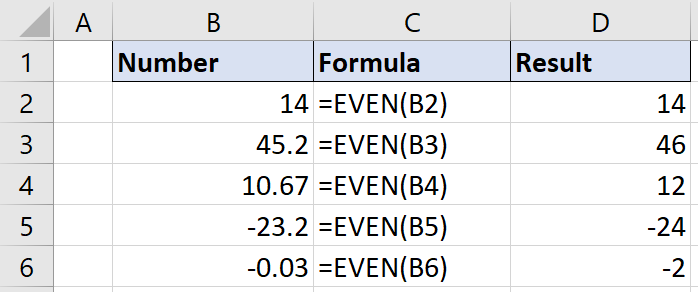## Extra Method: Use the Excel Formatting Options

By using the formatting options of Excel, you can change the presentation of the number, without actually changing the number.

So you could hide the decimals, or present a value to the nearest thousand, but it will not affect the original number.

The simplest way to show or hide the decimals in a range of cells is to use the Increase Decimal and Decrease Decimal buttons on the Home tab.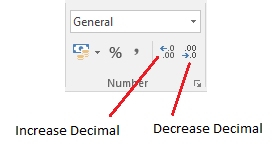When the decimals are hidden, Excel will round the values in the cell down for numbers 1-4, and up for numbers 5-9. This is just like the ROUND function, but does not change the value like ROUND.

Let’s look at another example. We can use the custom formatting options of Excel to display a number as thousands or millions.

1. Open the Format Cells dialog by pressing Ctrl + 1.
2. Ensure the Number tab is selected and then click Custom.
3. In the Type field enter 0, “K” to display as thousands or 0,, “M” to display as millions.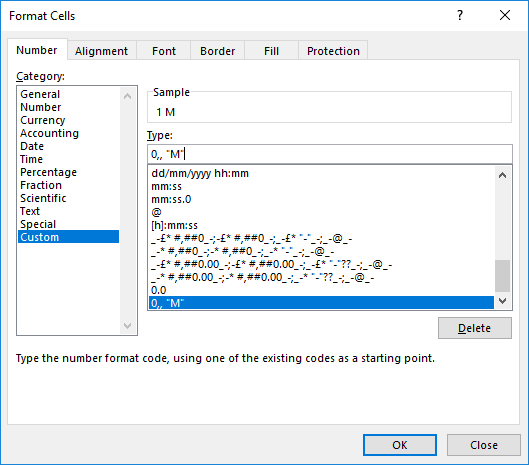The decimal places buttons can be used to show more detail if desired.

## Conclusion

Excel provides many ways to round values to a specific integer, or to a number of decimal places So whatever your requirements, Excel has a way.

The various rounding functions of Excel can be used to change and present the value in the best way possible. Be sure to understand the difference and choose the right one to prevent unexpected results.

Sometimes you may not want to affect the number, so the custom formatting feature of Excel is your ally in these moments.